Question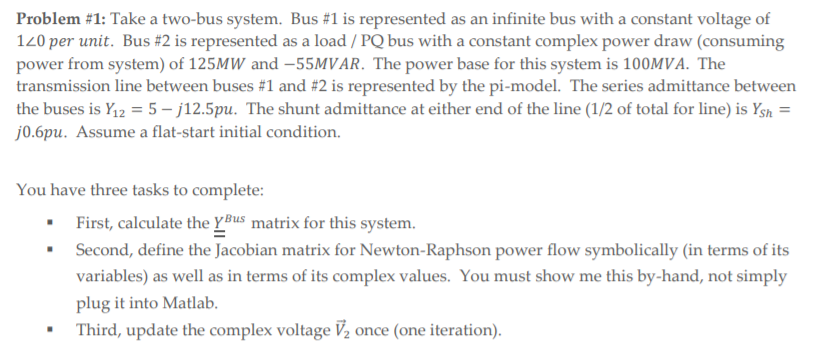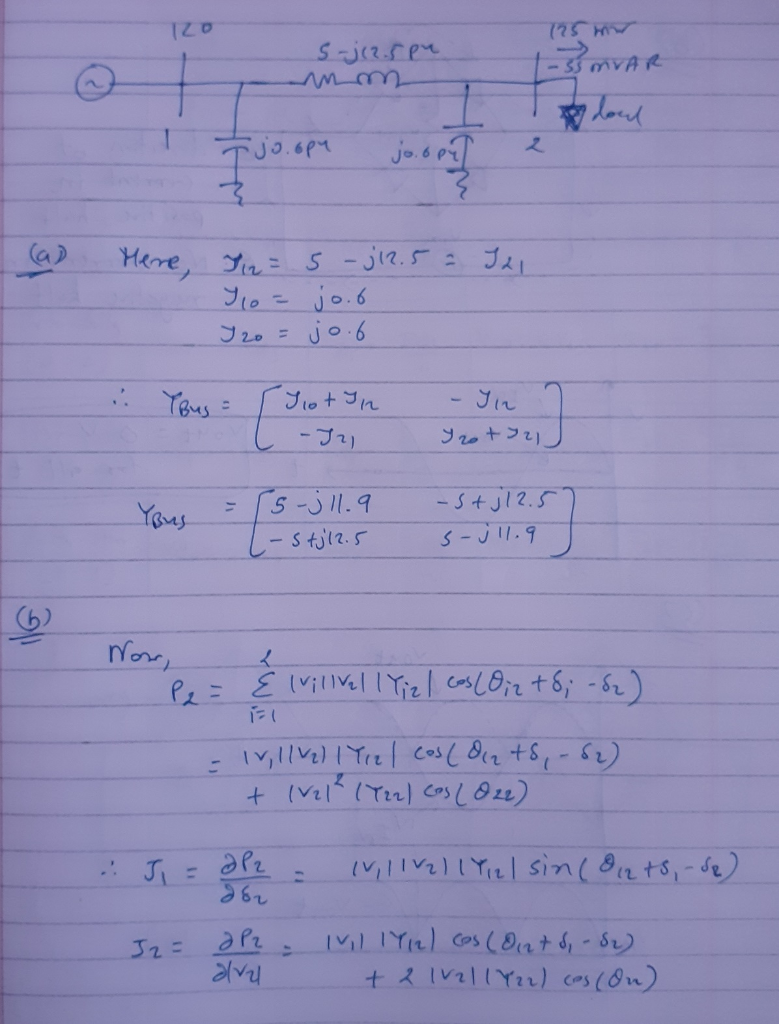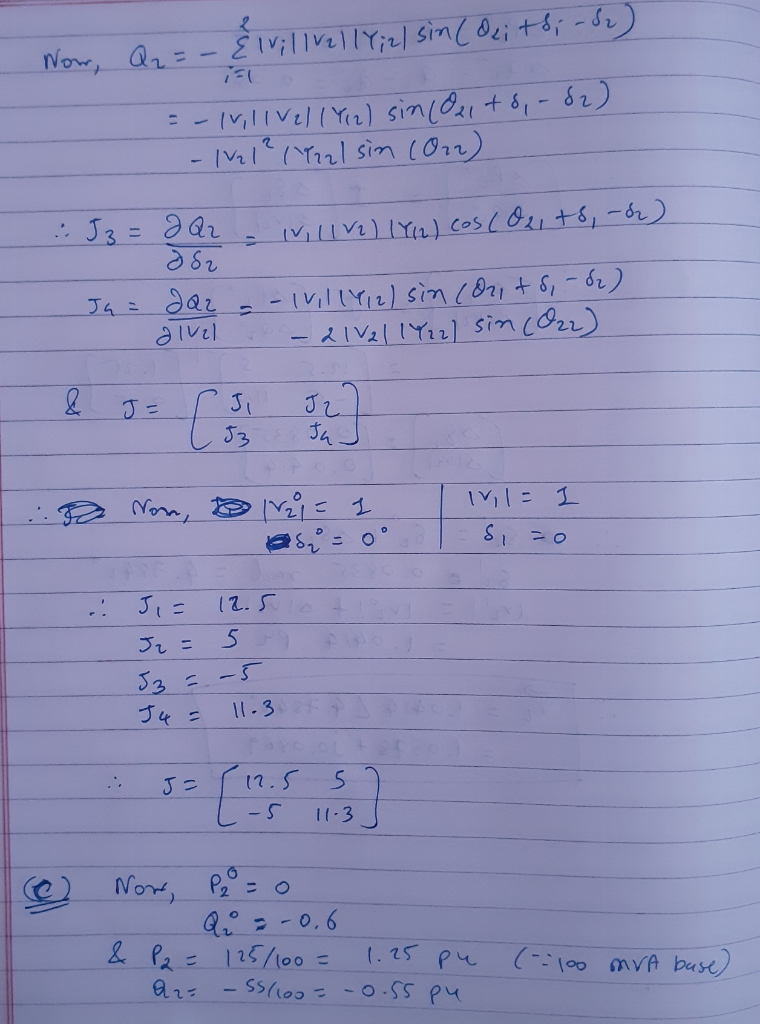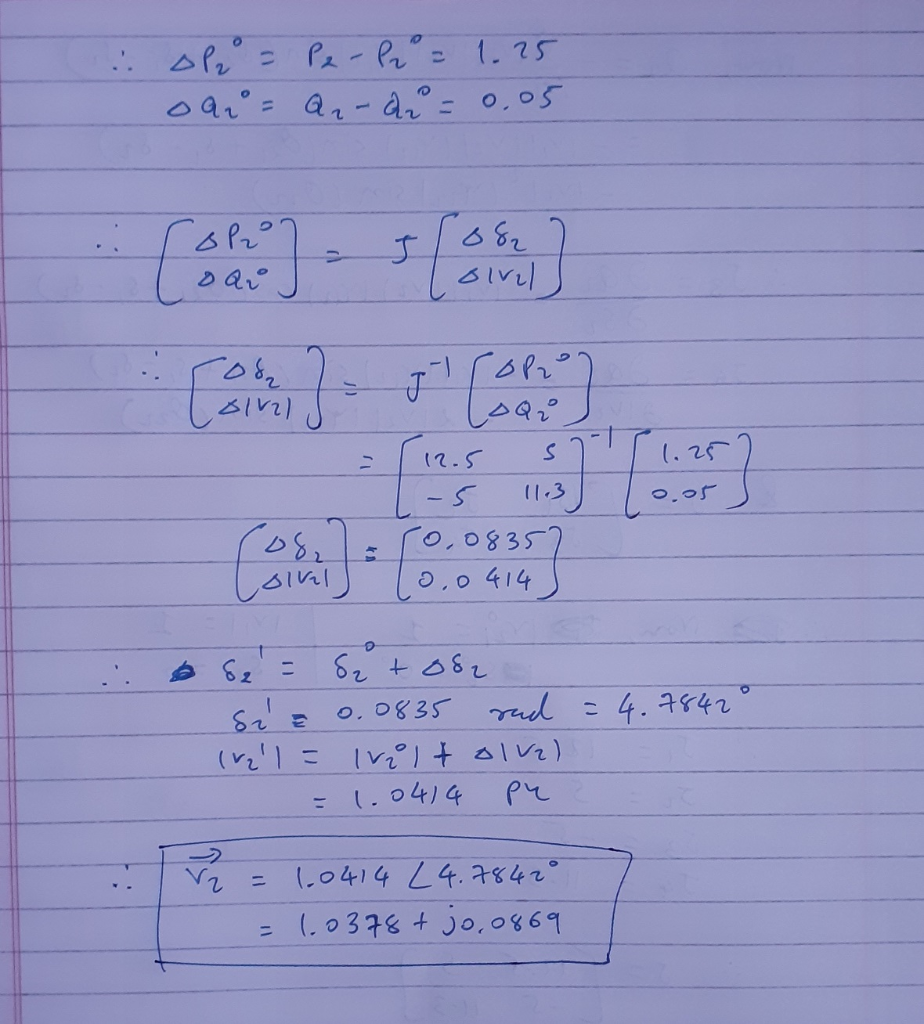#### Earn Coins

Coins can be redeemed for fabulous gifts.

Similar Homework Help Questions
• ### 3. (20pts) A balanced three phase twe-bu power system with S-100 MVA, V-100kv, is below Bus Bus 2 :150MVA @ pf0.8 lagging (1) Using the per unit analysis, formulate the Yes matrix (2) Determine t...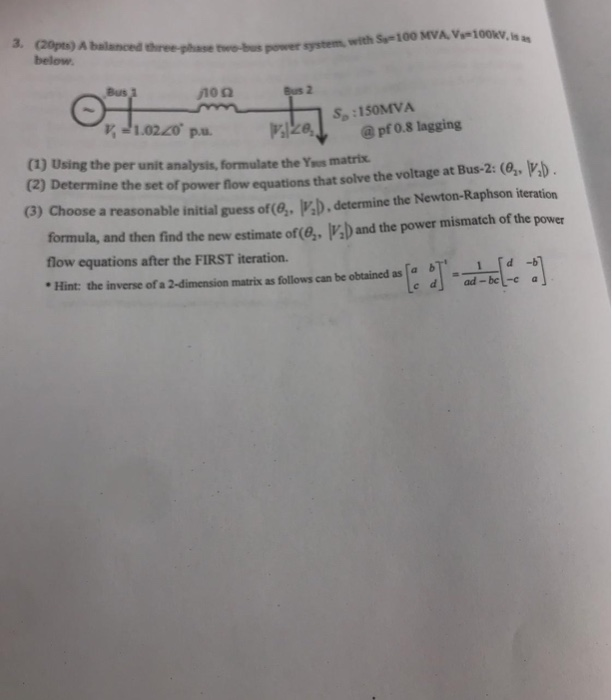3. (20pts) A balanced three phase twe-bu power system with S-100 MVA, V-100kv, is below Bus Bus 2 :150MVA @ pf0.8 lagging (1) Using the per unit analysis, formulate the Yes matrix (2) Determine the set of equations that equations that solve the voltage at Bus-2: (, D powver flow equations that solve the voltage at Bus-2: (e,, b G) Choose a reasonable initial guess of(e,. P'D. determine the Newton-Raphson iteration 's) and the power mismatch of the powe the...

• ### Q2 (Newton-Raphson Power Flow Solution with FACTS Devices Consider a two-bus system with the sing...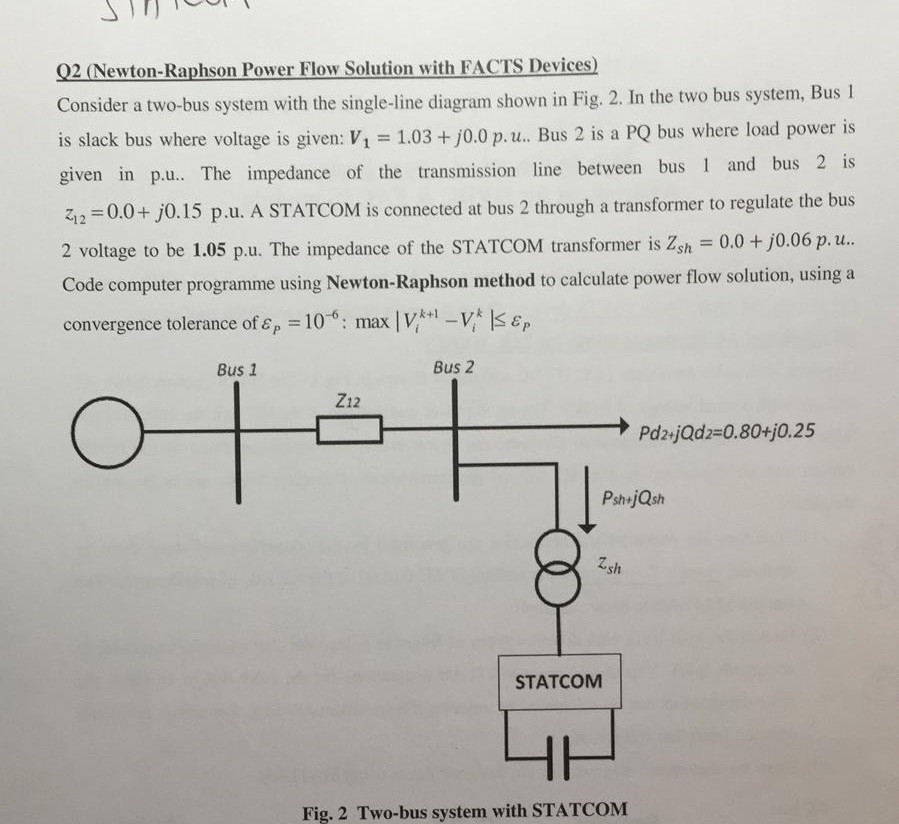do it in Matlab Q2 (Newton-Raphson Power Flow Solution with FACTS Devices Consider a two-bus system with the single-line diagram shown in Fig. 2. In the two bus system, Bus is slack bus where voltage is given: V 1.03 +j0.0 p. u. Bus 2 is a PQ bus where load power is given in p.u.. The impedance of the transmission line between bus 1 and bus 2 is 212-0.0+ j0.15 p.u. A STATCOM is connected at bus 2 through a...

• ### The single-line diagram of a three-phase live-bus power system is shown in Fig. 1. All lines...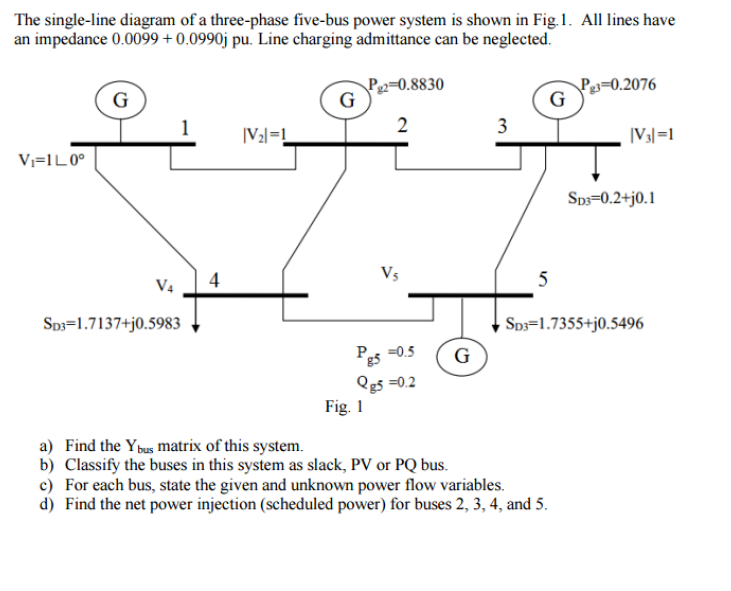The single-line diagram of a three-phase live-bus power system is shown in Fig. 1. All lines have an impedance 0.0099 + 0.0990j pu. Line charging admittance can be neglected. a) Find the Ybus matrix of this system. b) Classify the buses in this system as slack, PV or PQ bus. c) For each bus, state the given and unknown power how variables. d) Find the net power Injection (scheduled power) for buses 2, 3, 4, and 5.

• ### The single-line diagram of a three-phase five-bus power system is shown in Fig.1. All lines have ...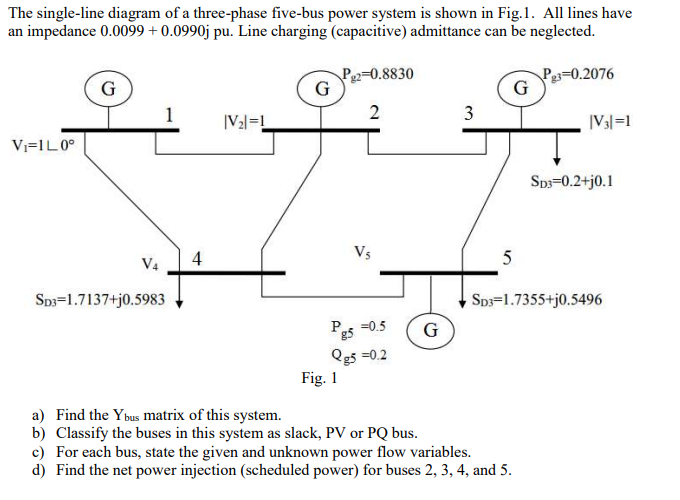The single-line diagram of a three-phase five-bus power system is shown in Fig.1. All lines have an impedance 0.0099+0.0990j pu. Line charging (capacitive) admittance can be neglected. 2-0.8830 30.2076 SD3-0.2+j0.1 SD3-1.7137+j0.5983 Sos-1.7355+j0.5496 Pe5 -0.5 G Qg5 -0.2 Fig. 1 a) Find the Ybus matrix of this system. b) Classify the buses in this system as slack, PV or PQ bus c) For each bus, state the given and unknown power flow variables. d) Find the net power injection (scheduled power)...

• ### Question1 A power system is shown in figure below. The generators at buses 1 and 2...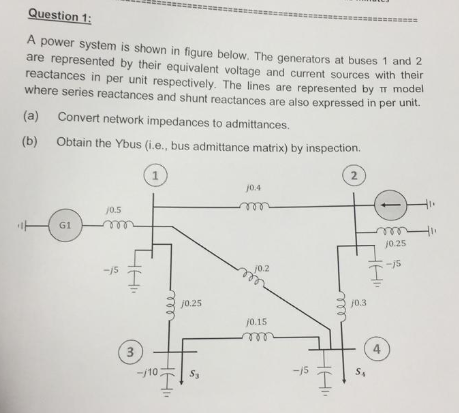Question1 A power system is shown in figure below. The generators at buses 1 and 2 are represented by their equivalent voltage and current sources wh their reactances in per unit respectively. The lines are represented by π model where series reactances and shunt reactances are also expressed in per unit. (a) Convert network impedances to admittances. (b) Obtain the Ybus (i.e., bus admittance matrix) by inspection. 0.4 /0.5 G1 0.2 0.25 0.3 0.15 4

• ### Q2. i) The one-line diagram of simple three-bus power system with generation at bus 1 is shown in figure Q2. 0.02 + 30.04 2 256.6 MW 0.0125 + 30.025 +110.2 Mvar 0.01 + 30.03 Slack Bus 3 Vi = 1.0520° 138.6 MW 45.2 Mvar Figure Q2 The magnitude of voltage at

Q2. i) The one-line diagram of simple three-bus power system with generation at bus 1 is shown in figure Q2. 0.02 + 30.04 2 256.6 MW 0.0125 + 30.025 +110.2 Mvar 0.01 + 30.03 Slack Bus 3 Vi = 1.0520° 138.6 MW 45.2 Mvar Figure Q2 The magnitude of voltage at bus 1 is adjusted to 1.05 per unit. The scheduled loads at buses 2 and 3 are as marked on the diagram. Line impedances are marked in per unit...

• ### BUS 1 BUS 2 Line Line 2 Line 3 Line 4 BUS 3 BUS 4 4x 4 bus admittance matrix for the above power system Generator activ...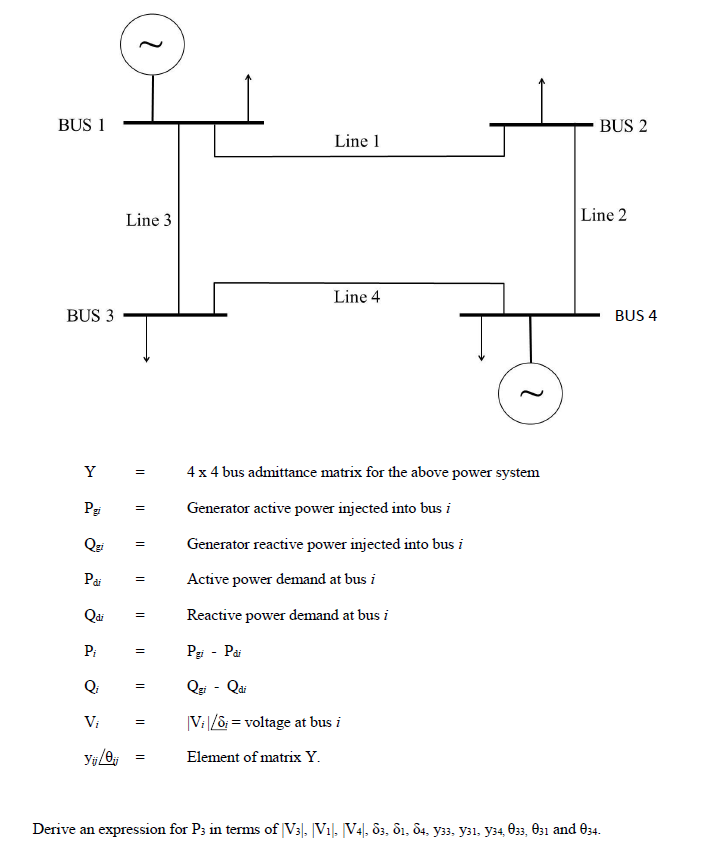BUS 1 BUS 2 Line Line 2 Line 3 Line 4 BUS 3 BUS 4 4x 4 bus admittance matrix for the above power system Generator active power injected into bus i Generator reactive power injected into bus i Active power demand at bus i Pa Reactive power demand at bus i Pg Pa Qi- Qai voltage at bus i Vi Vi Element of matrix Y expression for Ps in terms of V3.V1 V4, , 1., 84, y33. y31. y34,...

• ### 3- The figure given below shows the one line diagram of a simple power system. Line impedançe is given in per unit...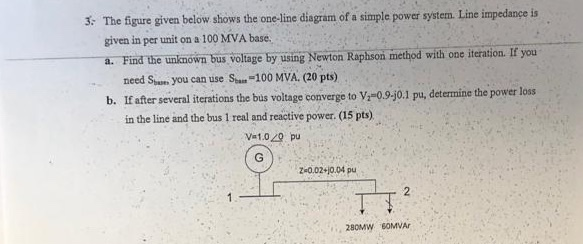3- The figure given below shows the one line diagram of a simple power system. Line impedançe is given in per unit on a 100 MVA base. a. Find the unknown bus voltage by using Newton Raphson method with one iteration. IE you need Ssum you can use San-100 MVA. (20 pts) b. If after several iterations the bus voltage converge to V09-j0.1 pu, determine the power loss in the line and the bus 1 real and reactive power. (15...

• ### The six-bus system shown in Figure 1 will be simulated using MATLAB. Transmission line data and b...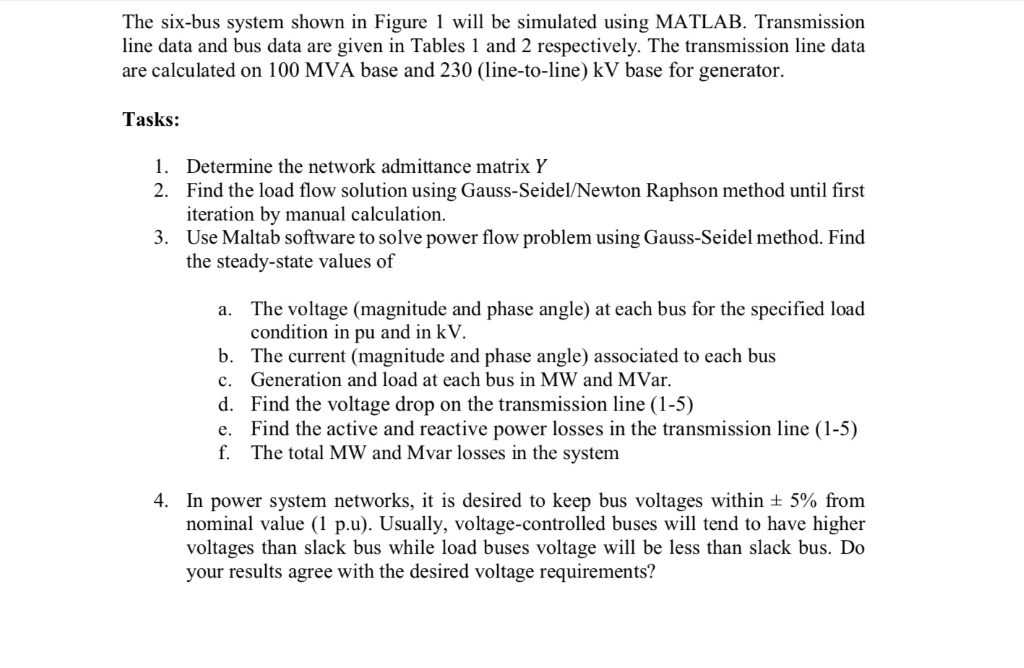The six-bus system shown in Figure 1 will be simulated using MATLAB. Transmission line data and bus data are given in Tables 1 and 2 respectively. The transmission line data are calculated on 100 MVA base and 230 (line-to-line) kV base for generator. Tasks: 1. Determine the network admittance matrix Y 2. Find the load flow solution using Gauss-Seidel/Newton Raphson method until first iteration by manual calculation. Use Maltab software to solve power flow problem using Gauss-Seidel method. Find the...

• ### Consider the 4-bus power system shown in Fig. 1. The system parameters are given below: 50 MVA, 2...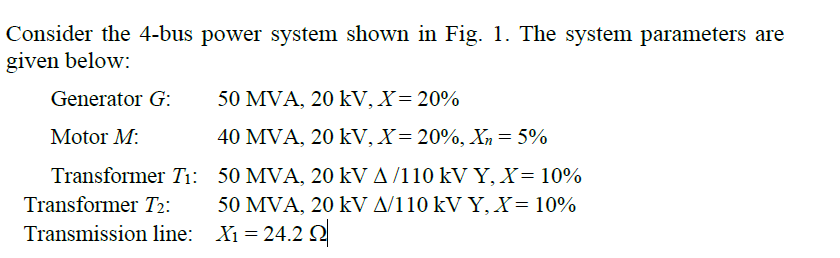Please show all the clearly step Y11 ist j30 and Y44 isnt -j12.85 Consider the 4-bus power system shown in Fig. 1. The system parameters are given below: 50 MVA, 20 kV, X-2090 40 MVA, 20 kV, X-20%, X, = 5% 50 MVA, 20 kV Δ /110 kV Ý, X= 1090 50 MVA, 20 kV MI 10 kV Ý, X= 10% Xi-24.2 Ω Generator G: Motor M: Transformer T1 : Transformer T2 : Transmission line: 3 4 T2 nu)M Fig....